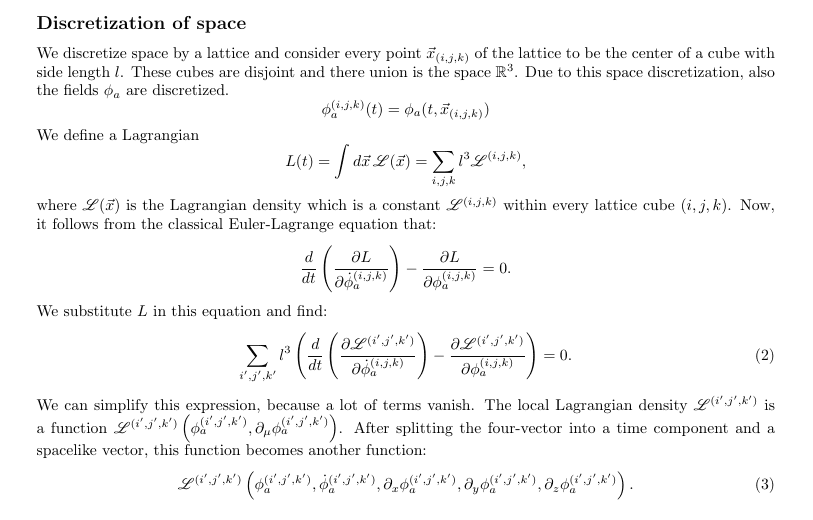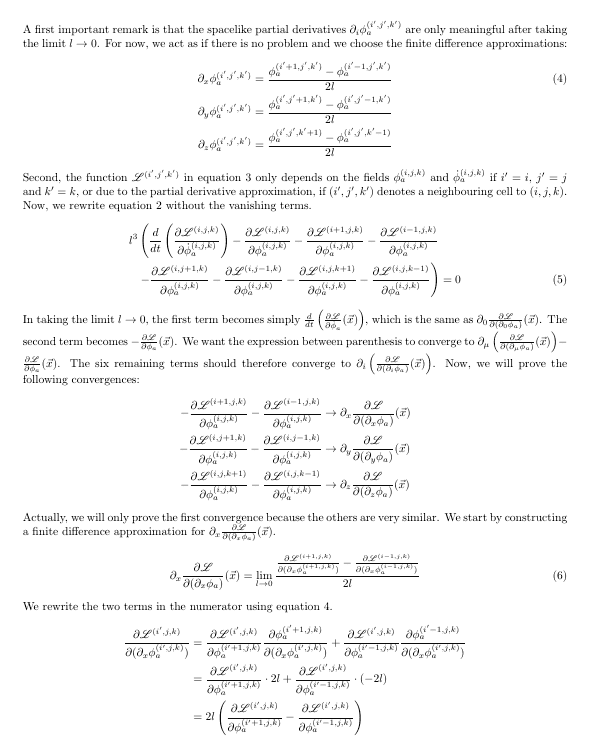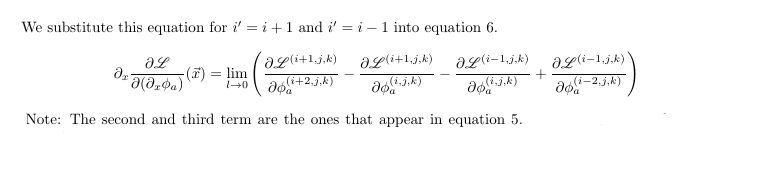# Euler Lagrange equations in continuum

JD_PM
Homework Statement:
Given

$$\frac{d}{dt} \frac{\partial L}{\partial \dot \phi_a^{(i j k)}} - \frac{\partial L}{\partial \phi_a^{(i j k)}} = 0 \tag{1}$$

Show that, in the limit ##l \rightarrow 0##, we obtain

$$\partial_{\mu} \frac{\partial \mathscr{L}}{\partial(\partial_{\mu} \phi_a)} - \frac{\partial \mathscr{L}}{\partial \phi_a} = 0 \tag{2}$$
Relevant Equations:
OK I've been stuck for a while in how to derive ##(1)##, so I better solve a simplified problem first:

We work with

Where

$$\mathscr{L} = \mathscr{L}(\phi_a (\vec x, t), \partial_{\mu} \phi_a (\vec x, t)) \tag{3}$$

And ##(3)## implies that ##\mathscr{L}(\vec x, t)##

We know that

$$L=l^3\sum_{(i j k)} \mathscr{L}^{(i j k)}(t) \tag{4}$$

Where

$$\lim_{l \rightarrow 0} L = \int d^3 \vec x \mathscr{L} \tag{5}$$

So, in analogy with ##(3), \mathscr{L}^{(i j k)}## depends on the fields ##\phi_a^{(i j k)} (t)##, on the time derivative of the fields ##\dot \phi_a^{(i j k)} (t)## and on the partial derivative of the fields with respect to ##x, y## and ##z## i.e;

$$\frac{\phi^{(i+1, j, k)}(t)-\phi^{(i, j, k)}(t)}{l} \tag{6.1}$$

$$\frac{\phi^{(i, j+1, k)}(t)-\phi^{(i, j, k)}(t)}{l} \tag{6.2}$$

$$\frac{\phi^{(i, j, k+1)}(t)-\phi^{(i, j, k)}(t)}{l} \tag{6.3}$$

Let's tackle the problem.

I would naively plug ##(4)## into ##(1)## and evaluate the terms to get

$$\frac{\partial}{\partial \dot \phi_a^{(i j k)}} \sum_{(i'j'k')} \Big[ l^3 \mathscr{L}^{(i' j' k')}(t) \Big] = l^3 \frac{\partial \mathscr{L}^{(i j k)}}{\partial \dot \phi_a^{(i j k)}} \tag{7.1}$$

$$\frac{\partial}{\partial \phi_a^{(i j k)}} \sum_{(i'j'k')} \Big[ l^3 \mathscr{L}^{(i' j' k')}(t) \Big] = l^3 \frac{\partial \mathscr{L}^{(i j k)}}{\partial \phi_a^{(i j k)}} \tag{7.2}$$

Where I've used the Kronecker delta.

Next I'd take the limit ##l \rightarrow 0##\$ of ##\frac{\partial \mathscr{L}^{(i j k)}}{\partial \dot \phi_a^{(i j k)}}## and ##\frac{\partial \mathscr{L}^{(i j k)}}{\partial \phi_a^{(i j k)}}## to get ##\frac{\partial \mathscr{L}}{\partial \dot \phi_a}## and ##\frac{\partial \mathscr{L}}{\partial \phi_a}## (respectively)

So I get

$$l^3 \Big( \frac{d}{dt} \frac{\partial \mathscr{L}}{\partial \dot \phi_a} - \frac{\partial \mathscr{L}}{\partial \phi_a} \Big) = 0 \tag{8}$$

Which of course does not yield ##(2)## when taking the limit ##l \rightarrow 0##.

The issue is that I am missing the spatial components ##(6.1), (6.2), (6.3)##...

I've been discussing this problem and related but we did not manage to really understand it.

Any help is really appreciated.

Thank you•Delta2

JD_PM
Alright here's another attempt (after discussing with a colleague).So it seems we are closer but we still do not see why certain terms in above's EQ. 5 should vanish when taking the limit ##l \rightarrow 0##.

The issue we really have is that we do not fully understand how to deal with discretized terms...

@samalkhaiat , would you have time for this?•etotheipi